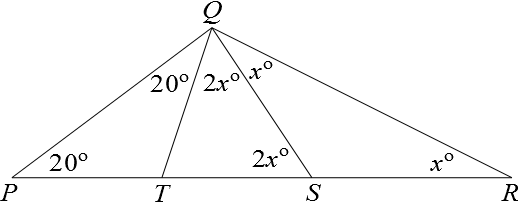#### You may also like### Isosceles

Prove that a triangle with sides of length 5, 5 and 6 has the same area as a triangle with sides of length 5, 5 and 8. Find other pairs of non-congruent isosceles triangles which have equal areas.Four rods, two of length a and two of length b, are linked to form a kite. The linkage is moveable so that the angles change. What is the maximum area of the kite?### Estimating Angles

How good are you at estimating angles?

# Tent Poles

##### Age 11 to 14 Short Challenge Level:

As $QS=SR$, triangle $SQR$ is isosceles, so $\angle SRQ= \angle SQR =x^{\circ}$.
So by the exterior angle theorem $\angle QST=2x^{\circ}$.

Also, $\angle TQS = 2x^{\circ}$ since $QT=TS$.

As $PT=QT$, $\angle TPQ=\angle TQP =20^{\circ}$.Since the interior angles of triangle $PQR$ must sum to $180^{\circ}$ we obtain $$20+(20+2x+x)+x=180$$ $$40+4x=180$$ $$4x=140$$ $$x=35.$$
This problem is taken from the UKMT Mathematical Challenges.
You can find more short problems, arranged by curriculum topic, in our short problems collection.